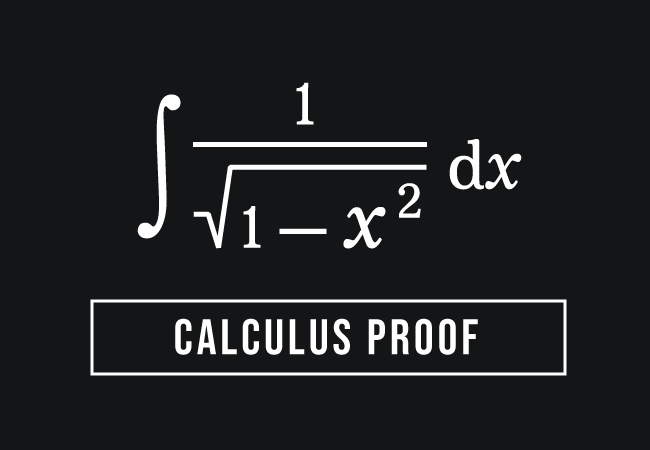# Calculus Proof of $\displaystyle \int{\dfrac{1}{\sqrt{1-x^2}}\,}dx$ RuleThe indefinite integration rule for one by square root of one minus square of a variable can be derived mathematically in calculus.

$\displaystyle \int{\dfrac{1}{\sqrt{1-x^2}}\,\,}dx$

In this case, the variable is denoted by $x$. So, the integral for this irrational function in algebraic form has to evaluate with respect to the same variable.

Now, let’s learn how to derive it in calculus.

### Derivative of Inverse sine function

The inverse sine function is written as $\sin^{-1}{(x)}$ or $\arcsin{(x)}$ mathematically in inverse trigonometry. According to the differential calculus, the derivative of the inverse sine function with respect to $x$ can be written in the following two forms.

$(1).\,\,\,$ $\dfrac{d}{dx}{\,\big(\sin^{-1}{x}\big)}$ $\,=\,$ $\dfrac{1}{\sqrt{1-x^2}}$

$(2).\,\,\,$ $\dfrac{d}{dx}{\,\big(\arcsin{x}\big)}$ $\,=\,$ $\dfrac{1}{\sqrt{1-x^2}}$

### Inclusion of an Arbitrary constant

The derivative of a constant is zero as per the differentiation. Hence, it does not influence the result when an arbitrary constant is added to the inverse sine function. Therefore, the above mathematical equation can be written as follows by adding a constant $c$ to sine inverse function.

$\implies$ $\dfrac{d}{dx}{\,\big(\sin^{-1}{x}+c\big)}$ $\,=\,$ $\dfrac{1}{\sqrt{1-x^2}}$

### Integral of Inverse sine function

The collection of all primitives of the quotient of one by square root of one minus square of a variable $x$ is called the indefinite integral of the quotient of one by square root of one minus square of a variable $x$. It is written in mathematical form as follows.

$\displaystyle \int{\dfrac{1}{\sqrt{1-x^2}}\,\,}dx$

An anti-derivative or the primitive of $1$ by square root of $1$ minus $x$ squared is the sum of the sine inverse of $x$ and the integral constant as per the fundamental relation between the integration and differentiation.

$\dfrac{d}{dx}{\,\big(\sin^{-1}{x}+c\big)}$ $\,=\,$ $\dfrac{1}{\sqrt{1-x^2}}$ $\,\Longleftrightarrow\,$ $\displaystyle \int{\dfrac{1}{\sqrt{1-x^2}}\,\,}dx$ $\,=\,$ $\sin^{-1}{x}+c$

Therefore, the integral of the multiplicative inverse of the square root of one minus $x$ squared is the inverse sine function plus the integral constant.

$\therefore\,\,\,$ $\displaystyle \int{\dfrac{1}{\sqrt{1-x^2}}\,\,}dx$ $\,=\,$ $\sin^{-1}{x}+c$

#### Alternative method

It can also be derived in a trigonometric method. So, let’s learn how to prove the integration of the one by square root of one minus $x$ squared by the trigonometric functions.

A best free mathematics education website that helps students, teachers and researchers.

###### Maths Topics

Learn each topic of the mathematics easily with understandable proofs and visual animation graphics.

###### Maths Problems

A math help place with list of solved problems with answers and worksheets on every concept for your practice.

Learn solutions

###### Subscribe us

You can get the latest updates from us by following to our official page of Math Doubts in one of your favourite social media sites.